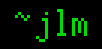## 29-Jun-2010

### Misuse of conditional probability

Filed under: math — jlm @ 13:27

When explaining conditional probability, the “two child” puzzle is often brought in, which is too bad because it’s a terrible example. Case in point, this recent blog post by Keith Devlin.

I tell you I have two children and that (at least) one of them is a boy, and ask you what you think is the probability that I have two boys.

(In a model where children’s sexes are independent and equiprobable,) he applies conditional probability to calculate the probability of them being the same sex at 1/3, in contrast to the “wrong” “intuitive” answer of 1/2. Let’s run with this 1/3 answer for a bit. The same analysis will show that if he tells us that one is a girl, the probability that the other is a girl is 1/3. He’s going to tell us either that he has a boy kid or he has a girl kid, and in both cases the probability of both children being the same sex is 1/3, so the unconditional probability of his children being same sex is 1/3, which is crazy. Where’d we go wrong?

The problem in the analysis is that him telling us he has a boy is not the same as conditioning the probability space on him having a boy. If you like calculating conditional probabilities, the proper condition to apply when he tells us he has a boy isn’t “he has a boy” but “he tells us he has a boy”. For the puzzle, we can assume he’ll be honest, so if he has two boys he’ll say he has a boy, and for two girls he’ll say he has a girl. The interesting case is if his children are mixed. Maybe he’ll say “boy” and “girl” half the time each in that case — but then, the probability of two boys turns out to be the intuitive 1/2 after all. Maybe he’ll always say “boy” then — this gives 1/3 for the quoted puzzle, as it “should”, but having the boy always trump the girl is unappealing and it’d give a 100% chance for two girls if he says he has a girl.

More importantly though, conditional probability is just trotted out there, with no consideration if it’s appropriate to the model. Is it appropriate? In the puzzle (“I tell you I have two children and that (at least) one of them is a boy, and ask you what you think is the probability that I have two boys.”) a reasonable model is he picks a kid of his at random and tells us its gender. He’d say he has a boy 50% of the time, and half of that time his other kid will also be a boy, as the kids’ genders are independent. Conditional probability doesn’t enter into this model. What would be the model where conditional probability was appropriate? It’s hard to come up with one which matches the wording of the puzzle, which is why I think using this puzzle for showing how conditional probability works is a mistake.

Keith Devlin’s article goes on to analyze the new “Tuesday birthday” puzzle:

I tell you I have two children, and (at least) one of them is a boy born on a Tuesday. What probability should you assign to the event that I have two boys?

once again blindly trotting out conditional probability. But let us first ask ourselves if it’s appropriate, what the proper model is. The least surprising model IMHO is that he picks one of his kids at random and tells us that child’s day-of-week and gender. In this model, independence between the children again applies and the probability of two boys is 1/2. What model could have conditional probability apply? Conditional probability applies when the other possibilities in the probability space are removed from consideration, so that’d be something like… a majordomo at a large gathering of parents of two children flips a coin to choose a gender (boy) and spins a spinner to select a day of week (Tuesday), sends away all the parents who don’t have a Tuesday-born son, and selects one of the remaining to tell you that they have a Tuesday-born son. The conditional probability of a second son is indeed 13/27, but models where conditional probability applies to the puzzle are farfetched, so applying conditional probability is an error. In this puzzle intuition is correct after all, the proper answer is 1/2.

[Update: Keith Devlin has pre-emptively addressed some of my criticism in his next post, The Problem with Word Problems, by saying that “I tell you X” is word-problem code for “the probability space is conditioned on X”. I still don’t like equating conditioning on “X” with conditioning on “he said X”: As above, if you have “One of my two kids is a boy, therefore the probability of my children being the same sex is 1/3.” then you can’t have “One of my two kids is a girl, therefore the probability of my children being the same sex is 1/3.”. I find the embrace of this asymmetry absurd.]

## 1 Comment

1. This is exactly why I found many explanations of the “Monty and the goats” problem lacking. They assume (usually without saying so) that Monty will only ever show you a door you didn’t pick that doesn’t have the prize behind it. While real-world Montys have an incentive to prolong the game and pump up the drama in this manner, I suspect sometimes the stage manager signals them to “wrap it up”, in which case they might just open the door you picked, or the one the prize is behind. You can’t calculate expected payoffs for your choices without assigning probabilities to Monty’s alternatives.

Comment by Jeremy Leader — 29-Jun-2010 @ 17:02

Sorry, the comment form is closed at this time.